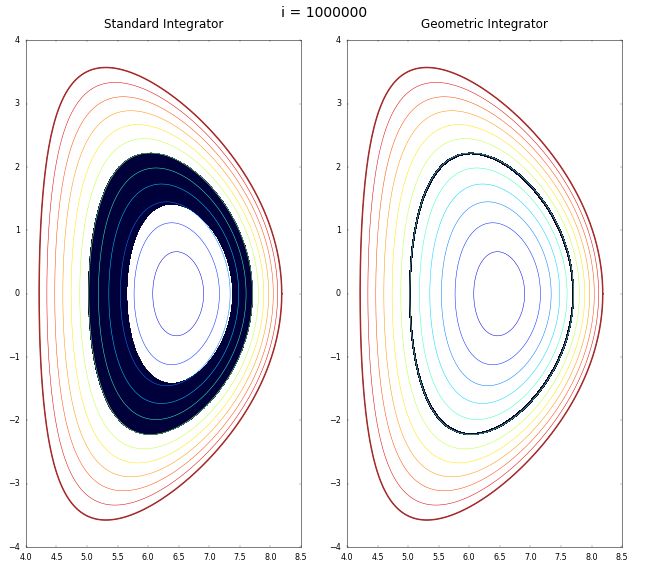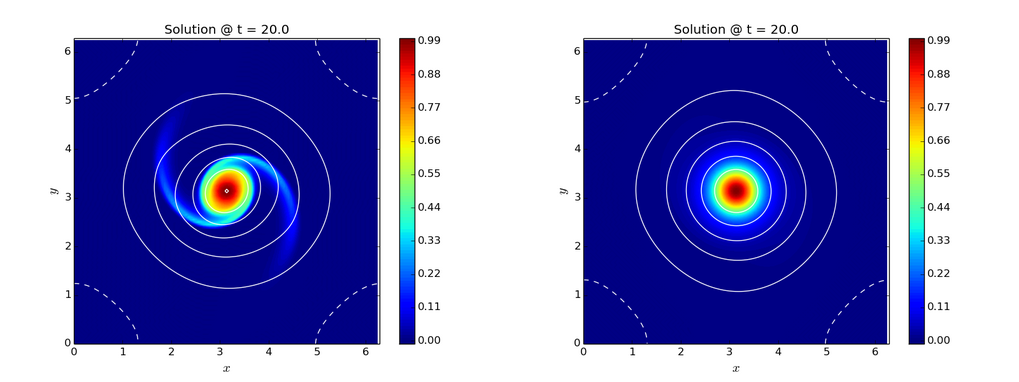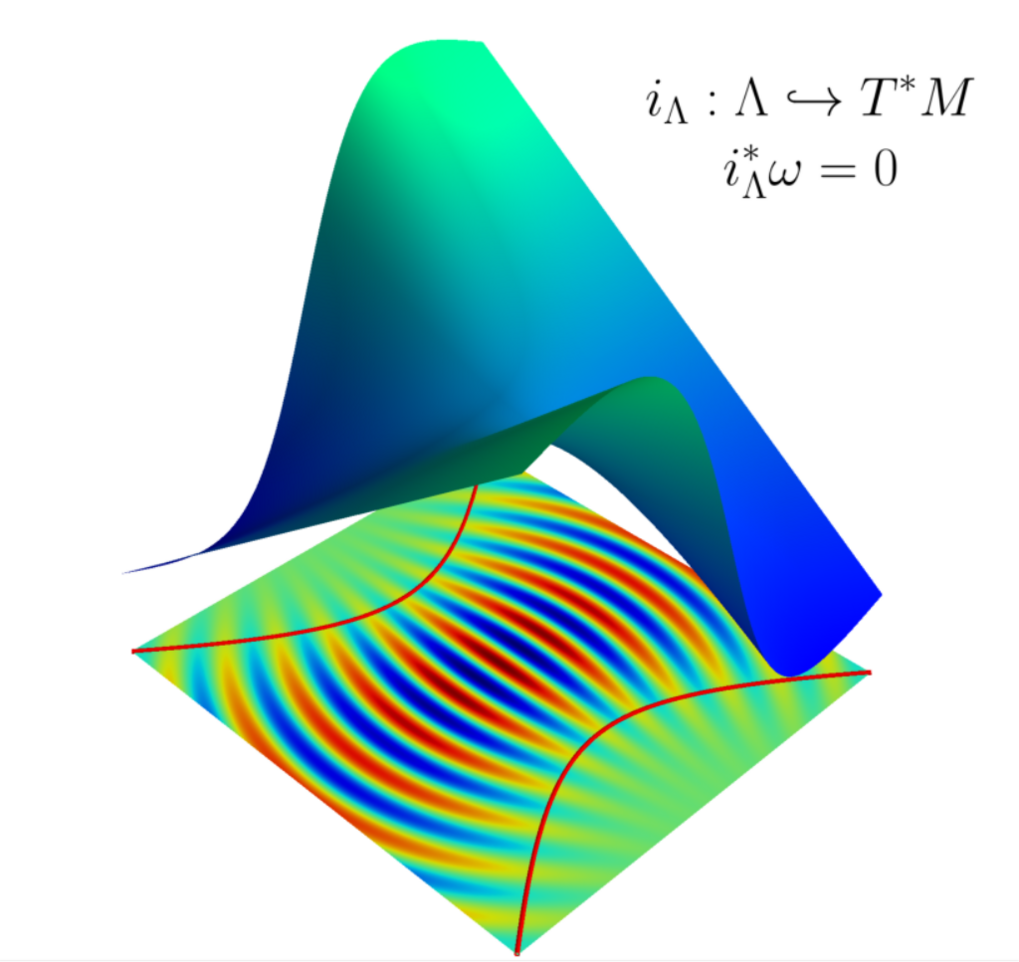Geometric and structure preserving methods

The geometry group of the NMPP division studies the abstract mathematical structures underlying plasma-physics models in order to design numerical algorithms that respect important qualitative properties of the physical problem.

Mathematical models in plasma physics are often extremely complex, reflecting the richness of plasma behaviour in nuclear fusion devices as well as in space and astrophysical plasmas. This is particularly true when such models are required to be physically realistic including full details on the geometry of the domain, boundary conditions and physics processes.

At a higher level of mathematical abstraction, such complicated models frequently reveal a rich and elegant "structure", which can often be understood in terms of modern Geometric Mechanics. By means of structure preserving methods, such mathematical structures are exploited in order to obtain qualitatively better numerical solutions that respect the physical conservation laws, devise new efficient algorithms for problems that are either not amenable to or extremely slow with standard discretization techniques, and suggest better formulations of reduced models that respect the structure of the original model.

Geometric Numerical IntegrationComparison between standard Runge-Kutta and geometric integrator for charged particle motion in an ITER-like Solov'ev equilibrium. While the geometric integrator describes the particle orbit correctly for thousands of periods, the standard integrator results in a monotonic decay of the orbit.

Many energy-preserving models of plasma physics amount to Hamiltonian dynamical systems, where the Hamiltonian function is usually given by the energy itself and the dynamics is generated by appropriate Poisson brackets. This is the case of both ideal magnetohydrodynamics and the Vlasov-Maxwell system. Preserving the Hamiltonian structure at the discrete level guarantees good qualitative properties of the numerical solution like error bounds on important quantities such as energy.

Many models of plasma physics also exhibit a variational structure, which can be discretized by variational integrators. This leads to numerical methods which satisfy important conservation laws, like momentum conservation, exactly and reproduce the qualitative correct physical behaviour also in long-time simulations. The application of variational integrators to both plasma particle dynamics (ordinary differential equations) and plasma field theories (partial differential equations) requires an appropriate extension of the theory in order to deal with important plasma-physics models such as, guiding centre dynamics, magnetohydrodynamics and kinetic physics.

MHD Equilibrium and Steady-State Transport

Mathematical dynamical systems that maximize entropy functionals can be constructed in oreder to obtain steady-state solutions of physical models quickly. Such dynamical systems are obtained either from the underlying Hamiltonian structure when available, or by ad-hoc mechanisms. Applications include magnetohydrodynamics equilibria and steady-state transport models.Vorticity equation in two dimensions. Left: Ideal dynamics. Right: Metriplectic dynamics. The metriplectic dynamics quickly evolves the fluid towards an equilibrium of the ideal dynamics.

Finite Element Analysis of Saddle-Point Problems

Another example of occurring mathematical "structure" that do not necessarily fall into the Geometric Mechanics category is found in saddle-point problems for transport equations with constraints. Finite element analysis of such saddle-point problems consitutes a new approach to the problem of determining the self-consistent electrostatic potential occurring in the physics of the plasma edge.

Semiclassical Asymptotics and Microlocal MethodsContours of the wave field of a paraxial beam on the two-dimensional plane together with a three-dimensional representation of the associated Lagrangian manifold. The Lagrangian manifold is a submanifold of the four-dimensional phase space that can be viewed as a geometric representation of the phase of the wave field.

Hamiltonian mechanics plays an important role in the description of high-frequency wave beams in fusion plasmas. In this case the Hamiltonian structure is "hidden" and can be exposed by application of either semiclassical or microlocal techniques. Numerical codes based upon these techniques are at the same time light-weight and performant while retaining the essential physics of the wave propagation. Applications include heating, current-drive and diagnostic systems in magnetic fusion devices.

Numerical Codes

• WKBeam
Monte-Carlo solver for electron-cyclotron wave beams developed in collaboration with IPP's tokamak theory division.

• GeometricIntegrators.jl
Library of geometric integrators for ordinary differential equations and differential algebraic equations in Julia.

• viIMHD2D, viRMHD2D, viVlasov1D
Reference implementations of variational integrators for ideal magnetohydrodynamics (viIMHD2D), reduced magnetohydrodynamics (viRMHD2D) and the Vlasov-Poisson system (viVlasov1D).
Go to Editor View